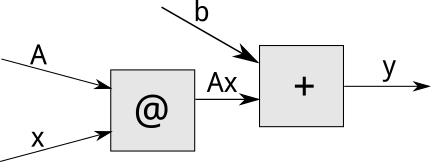# Rerun a computation graph

Hi,

suppose we have the following code:

``````A = torch.eye(2, requires_grad=True)
x = torch.Tensor([1, 2])
y = A@x + b  # y = tensor([2, 3])
``````

This instantiates (and automatically runs) a computation graph which looks something likeNow, suppose one of the leaf variables changes:

``````A.data[0, 0] = 3
``````

Now, if we wish to get the new value of `y`, we need to manually run all the calculations (I think this instantiates a new graph which looks exactly the same - but I may be wrong here):

``````y = A@x + b  # y = tensor([4, 3])
``````

Is there a more automatic way to recompute/rerun the computation graph after some of the elements have changed?

I know that, in principle, I could contain the whole process of computing `y` inside a single function - and then just call that function again after the change. The problem with this is that my actual application involves multiple passes through the network, each with different data, as well as multiple calls to `backward(create_graph=True)`, and so wrapping everything in a single function would cause a mess from the software architecture and code readability perspective.

So, I would be grateful if someone could point me to a cleaner way of rerunning an existing graph. The ideal solution here would be a function in line of `y.recompute_from_graph()`.

1 Like

Hi,

In pytorch, the graph is only defined by the code. So you need to run the code to get the new output. The whole framework is built such that reconstructing the graph is cheap enough that you can do it every time you need to compute an output and you won’t see the difference performance wise.

1 Like

My concerns were not really related to efficiency, but more to code readability, as my code is more complex than a “standard” supervised learning scenario (forward-backward-step-repeat).
I thought that - maybe - it would be possible to use the information stored in `grad_fn` to repeat the calculations without explicitly calling the original code, but it turned out that - after some careful architectural considerations - rerunning the method did not cause a readability disaster after all, so that’s what I ended up doing